## TN State Board 12th Computer Science Important Questions Chapter 16 Data Visualization Using Pyplot: Line Chart, Pie Chart and Bar Chart

Question 1.
What is Infographics?
An Infographic is the representation of information in a graphic format.

Question 2.
A Dashboard is a collection of resources assembled to create a single unified visual display.

Question 3.
What is Matplotlib?

• Matplotlib is the most popular data visualization library in python.
• It allows you to create charts in few lines of code.Question 4.
What is difference between Scatter plot and Box plot?

 Scatter plot Box plot A scatter plot is a type of plot that shows the data as a collection of points. The Box plot is a standerdized way of displaying the distribution of data based on the five number summary. The position of a point depends on its two dimensional value. Minimum, first quartile, median, third quartile and maximum.

Question 5.
What is called markers?
A line chart or line graph is a type of chart . which displays information as a series of data points called markers. It is connected by straight line segments.

Question 6.
What is pip?

1. Matplotlib installed using pip.
2. Pip is a management software for installing python package.Question 7.
What is the use of pan Axis?
Pan Axis is cross-looking button allows you to click it, and then click and drag your graph around.

Question 8.
What are different kinds of plot that allows Matplotlib?
Matplotlob allows you to crate different kinds of plots ranging from Histograms and scatter plots to bar graphs and bar charts.

Question 9.
How will you start to draw a chart?
(i) By importing Matplotlib using the command import matplotlib.pyplot as pit.
(ii) Now you have imported Matplotlib in your work space. You need to display the plots.
(iii) Using Matplotlib from within a python script.
(iv) Now you have to add plt.show() method inside the file to display your plot.
Eg:
import malplotlib.pyplot as pit plt.
plot ([1, 2, 3, 4])
plt.show( )

Output: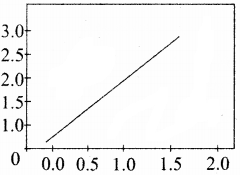Question 10.
What are the key difference between Histogram and Bar graph.
The differences between Histogram and bar graph are as follows:

 Histogram Bar Graph Histogram refers to a graphical representation; that displays data by way of bars to show the frequency of numerical data. A bar graph is a pictorial representation of data that uses bars to compare different categories of data. A histogram represents the frequency distribution of continuous variables. A bar graph is a diagrammatic comparison of discrete variables. The histogram is drawn in such a way that there is no gap between the bars. On the other hand, there is proper spacing between bars in a bar graph that indicates discontinuity. Histogram presents numerical data. Bar garaph shows categorical data. Items of the histogram are numbers, which are categorised together, to represent ranges of data. As opposed to the bar graph, items are considered as individual entities. A histogram, this cannot be done, as they are shown in the sequence of classes. Bar graph, it is quite common to rearrange the blocks, from highest to lowest. The width of rectangular blocks in a histogram may or may not be same. The width of the bars in a bar graph is always same.Question 11.
Create a Bar chart on Range and Marks of plus two students.
import matplotlib.pyplot as pit
labels=[“TAMIL”, “ENGLISH”, “MATHS”, “PHYSICS”, “CHEMISTRY”, “CS”]
usage – [79.8, 67.3, 77.8, 68.4, 70.2, 88.5]
y_positions = range (len(labels))
plt.bar (y positions, usage) plt.xticks (y_positions, labels)
plt.ylabel (“RANGE”) plt.title (“MARKS”)
lt.show( )
Output: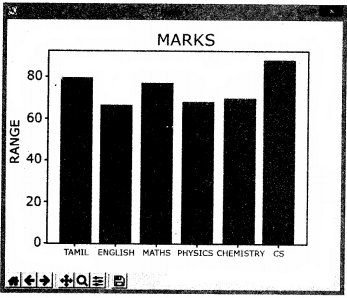Question 12.
Write a code to display the given plot, import matplotlib.pyplot as pit
x = [1,2,3]
y= [5,7,4]
x2 = [1,2,3]
y2 = [10,14,12]
plt.plot(x, y, label=’Line 1’)
plt.plot(x2, y2, label=’Line 2’)
plt.xlabel(‘X-Axis’)
plt.ylabel(‘Y-Axis’)
plt.title(‘LINE GRAPH’)
plt.legend( )
plt.show( )Question 13.
Write a code to display the following plot. import matplotlib.pyplot as pit.
plt.plot([1,2,3,4], [1,4,9,16])
plt.show( )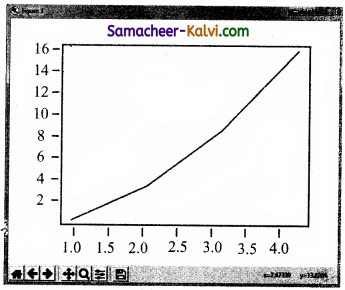Question 14.
Define: Data Visualization.
Data visualization is the graphical representation of information and data.
The data visualization uses statistical graphics, Numerical data may be enclosed using dots, lines or bars, to visually communicate a quantitative message.Question 15.
List the general types of data visualization.
General types of Data visualization are charts, Tables, Graphs, Maps, Infographics and dashboards.

Question 16.
List the types of Visualizations in Matplotlib.
There are many types of visualizations under matplotlib. Some of them are Line plot, Scatter plot, Histogram, Box plot, Bar chart and pie chart.

Question 17.
How will you install Matplotlib?

1. Install matplotlib using pip. Python – m pip install – u pip
2. Pip is a management software for installing python packages.
3. After installing matplotlib, we will begin coding importing matplotlib.Question 18.
Write the difference between the following functions: pltplot([1,2,3,4]), pit plot([1,2,3,4], [1,4,9,16]).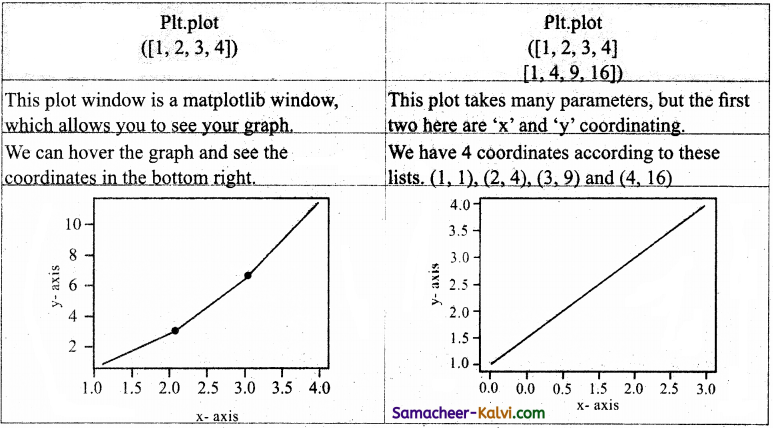Question 19.
Draw the output for the following data visualization plot.
import matplotlib.pyplot as pit
plt.bar([l,3,5,7,9],[5,2,7,8,2], label=”Example one’7)
plt.bar([2,4,6,8,10],[8,6,2,5,6], label=”Example two”, color=’g’)
pit.legend( )
plt.xlabel(‘bar number’)
plt.ylabel(‘bar height’)
plt.title(‘Epic Graph\nAnother Line! Whoa’)
plt.show( )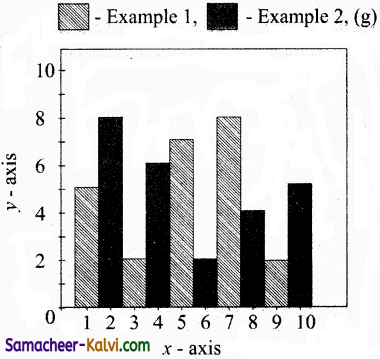Question 20.
Write any three uses of data visualization.
Uses of Data visualization:

1. Data visualization helps users to analyze and interpret the data easily.
2. It makes complex data understandable and usable.
3. Various charts in Data visualization 1 helps to show relationship in the data one or more.

Question 21.
Write the coding for the following:
(a) To check if PIP is Installed in your PC.
(b) To Check the version of PIP installed in your PC.
(c) To list the packages in matpiotlib.
(a) c:\users\yourName\AppData\Local\ program s\python\python3 6-3 2\ scripts>pip
(b) c:\users\yourName\AppData\Local\ programs\python\python36-32\ j scripts>pip – versions.
(c) c:\users\yourName\AppData\Local\ programs\python\python36-32\ scripts>pip — list

Question 22.
Write the plot for the following pie chart output.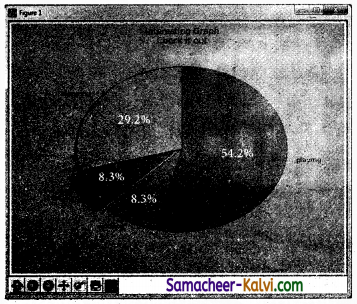import matplotlib.pyplot as pit
slices = [7, 2, 2, 13]
Activities = [‘Sleeping’, ‘Eating’, ‘Working’, ‘Playing’]
Cols = [‘c’, ‘m’, ‘r’, ‘b’]
plt.pie(slices, labels = Activities,
colors – cols
startangle = 90,
explode = (0, 0.1, 0, 0),
autopct = ‘% 1.1 f%%’),
Plt.title (“Interesting Graph \n check it out”)
pit. show ( )Question 23.
Explain in detail the types of pyplots using Matpiotlib.
Types of pyplots using matpiotlib:
Matpiotlib allows to create different kinds of j plots ranging from Histograms and scatter I plots to bar graphs and bar charts.
(i) Line chart:
A Line chart or Line Graph is a type of chart which displays information as a series of data points called ‘markers’ connected by straight line segments.
A line chart is often used to visualize a trend in data over intervals of time – a time series – thus the line is often drawn chronologically.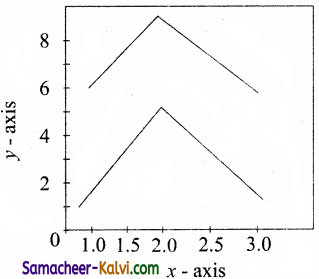(ii) Bar chart:
A Bar chart or Bar plot is one of the most common type of plot. It shows the relationship between a numerical variable and a categorical variable. Bar plot represents categorical data with rectangular bars.
Each bar has a height corresponds to the value it represents. The bar can be plotted vertically or horizontally.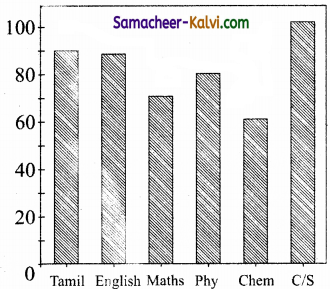Pie charts:
Pie chart is probably one of the most common type of chart.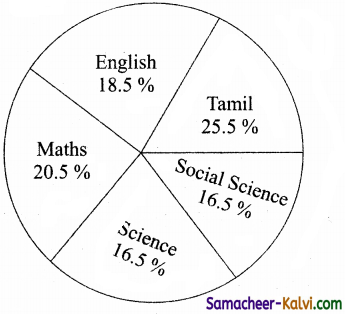It is a circular graphic which is divided into slices to illustrate numerical proportion.
To make a pie chart with matplotlib, we vcan use the plt.pie ( ) function.Question 24.
Explain the various buttons in a matplotlib window.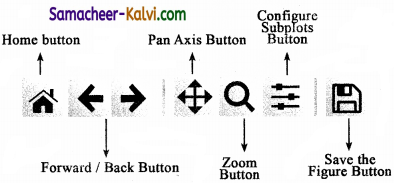Home Button → The Home Button will help once you have begun navigating your chart.
Forward/Back buttons → These buttons can be used like the Forward and Back buttons in your browser.
Pan Axis → This cross-looking button allows you to click it and then click and drag your graph around.
Zoom → The Zoom button lets you click on it, then click and drag a square that you would like to zoom into specifically. Zooming in will require a left click and drag. You can alternatively zoom out with a right click and drag.
Configure Subplots → This button allows you to configure various spacing options with
Save Figure → This button will allow you to save your figure in various forms.

Question 25.
Explain the purpose of the following functions:
(i) plt.xlabel (ii) plt.ylabel (iii) plt.title (iv) plt.legend( ) (v) plt.show ( )
(i) plt.xlabel – we can assign labels to those respective x-axis.
(ii) plt.ylabel – we can assign labels to those respective y-axis.
(iii) plt.title – we can assign the plot’s title, i.e., Heading of the plot.
(iv) pit.legend ( ) – This command, which automatically a legend for any labeled plot elements.
(v) plt.show ( ) – It will display the current figure that you are working on.Question 26.
Create a plot. Set the title, the x and y labels for both axes.
import matplotlib.pyplot as pit.
X = [1, 2, 3]
Y= [5, 7, 4]
plt.polt (x,y, label = ‘Linel’)
plt.xlabel (‘x-Axis’)
plt.ylabel (‘y-Axis’)
plt.title (‘LINE GRAPH’)
pit.legend ( )
plt.show ( )

Question 27.
Plot a pie chart for your marks in the recent examination.
import matplotlib.pyplot as plt
plt.style.use(‘gg plot’)/(‘pie plot’)
subject = (‘TAMIL’,‘ENGLISH’, ‘PHYSICS’, ‘CHEMISTRY’, ‘MATHS’, ‘COMP.SCIENCE’)
Marks = [78,79,95,96,98,99]
Pie-color = (“red”,“green”,“orange”,“cyan”, “blue”)
figl ax = plt.subplots( )
ax.pie(Subjects, Labels = Marks, autoplt = %l.If%%, “colors = Pie color, Starangle = 90)
ax.axis(equal)
ax.set_title(“MY RECENT MARKS\n”, Font size – 25)
plt.show( )Question 28.
Plot a linechart on the academic performance of Class 12 students in Computer Science for the past 10 years.
import matplotlib.pyplot as pit
Performance = [100,99,98,70,80,90,85,89, 60,75)
line_chart = plt.plot(range(50,100), Performance)
plt.title(“Academic Performance of class 12 students in Computer Science past 10 years”)
plt.xlabel(‘Performance’)
plt.ylabel(‘Years’)
plt.legend([Performance of Comp.Science’], 10c = 4)
plt.show( )

Question 29.
Plot a bar chart for the number of computer science periods in a week.
import matplotlib.pyplot as pyplot
from Tools.demo.sortview import WIDTH
from tortle demo.nim import color
pyplot.bar([‘MON’,‘TUE’,‘WED’, ‘THU’, ‘FRI’], WIDTH = 0.5,)
color = ([‘blue’,‘orange’,‘red’,‘green’])
pyplot.xlabel(“Periods in a week”)
pyplot.ylabel(“Days of week”)
pyplot.show( )Question 1.
Which is the representation of information in a graphic format?
(a) Infographic
(b) Dashboard
(c) Matplotlib
(d) Visual format
(a) Infographic

Question 2.
Which is a collection of resources assembled to create a single unified visual display?
(a) Infographic
(b) Dashboard
(c) Matplotlib
(d) Visual format
(b) Dashboard

Question 3.
Which is the most popular data visualization library in python?
(a) Infographic
(b) Dashboard
(c) Matplotlib
(d) Visual format
(c) MatplotlibQuestion 4.
Which is a type of plot that shows the data as a collection of points?
(a) Scatter plot
(b) Box plot
(c) Line plot
(d) Bar plot
(a) Scatter plot

Question 5.
Which is a standardized way of displaying the distribution of data based plot?
(a) Scatter plot
(b) Box plot
(c) Line plot
(d) Bar plot
(b) Box plot

Question 6.
Which method is used inside the file to display your plot?
(a) plt.show
(b) plt.legend
(c) plt.plot
(d) plt.title
(a) plt.showQuestion 7.
Which button allows you to click it and then click and drag your graph around?
(a) Home
(b) Pan Axis
(c) Zoom
(d) Back
(b) Pan Axis

Question 8.
Which chart displays information as a series ; of data points?
(a) Line
(b) Pie
(c) Histogram
(d) Bar
(a) Line

Question 9.
Which plot is one of the common type?
(a) Line
(b) Pie
(c) Histogram
(d) Bar
(d) BarQuestion 10.
Which plot is probably one of the most common type?
(a) Line
(b) Pie
(c) Histogram
(d) Bar
(b) Pie

Question 11.
Match the following:

 (i) Line chart A. two-dimension (ii) Bar chart B. auto pit (iii) Pie chart C. catergorical data (iv) Scatter plot D. markers

(a) (i) – D, (ii) – C, (iii) – B, (iv) – A
(b) (i) – D, (ii) – A, (iii) – B, (iv) – C
(c) (i) – B, (ii) – C, (iii) – A, (iv) – D
(d) (i) – B, (ii) – D, (Hi) – A, (iv) – C
(a) (i) – D, (ii) – C, (iii) – B, (iv) – A

Question 12.
Choose the incorrect pair:
(a) Infographic – Information
(b) Matplotlib – Library
(c) Dashboard – Multi plot
(d) Pip – Software
(c) Dashboard – Multi plotQuestion 13.
Choose the correct pair (Buttons):
(a) Home – begun
(b) PAN – Forward
(c) Zoom – drag
(d) Subplot – Back
(a) Home – begun

Question 14.
Choose the incorrect statement.
(a) Numerical data may be encoded using only pictures.
(b) Data visualization helps users to analyze and interpret the data easily.
(c) Complex data understandable and usable by using charts.
(d) Various charts in Data visualization help to show relationship in the data.
(a) Numerical data may be encoded using only pictures.

Question 15.
Choose the correct statement.
(a) Bar charts represent categorical data with rectangular bars.
(b) Bar chart can be plotted only vertically.
(c) The Box plot is a data based on the three number summary.
(d) Scatter plot is a point depend on its three dimensional value.
(a) Bar charts represent categorical data with rectangular bars.Question 16.
Assertion ( A):
Matplotlib is the most popular data visualization library in python.
Reason (R):
It allows you to create charts in few lines of code.
(a) Both A and R are True and R is the correct explanation for A.
(b) Both A and R are True but R is not the correct explanation for A.
(c) A is True but R is False.
(d) A is False but R is True.
(a) Both A and R are True and R is the correct explanation for A.

Question 17.
Assertion (A):
Histogram refers to a graphical representation of data.
Reason (R):
A Bar graph is a pictorial representation of data.
(a) Both A and R are True and R is the correct explanation for A.
(b) Both A and R are True but R is not the correct explanation for A.
(c) A is True but R is False.
(d) A is False but R is True.
(b) Both A and R are True but R is not the correct explanation for A.

Question 18.
Pick the odd one out.
(a) Line
(b) Scatter
(c) Box
(d) Zoom
(d) ZoomQuestion 19.
Matplotlib is a:
(a) Function name
(b) Reserved word
(c) Library
(d) Key word
(c) Library

Question 20.
Matplotlib allows to create a:
(a) graphics
(b) maps
(c) picture
(d) charts
(d) charts

Question 21.
How many types of visualizations under matplotlib?
(a) five
(b) six
(c) seven
(d) many
(d) manyQuestion 22.
Which is a type of plot that shows the data as a collection of points?
(a) Line plot
(b) Box plot
(c) Scatter plot
(d) Pie plot
(c) Scatter plot

Question 23.
Which plot is the distribution of data based on the five number summary?
(a) Line plot
(b) Box Plot
(c) Scatter plot
(d) pie plot
(b) Box Plot

Question 24.
Which command is used to import matplotlib for coding?
(a) import matplotlib.pyplot as pit
(b) import matplotlib.pypt as pt
(c) import matplotlb.pyplt as Pit
(d) import mathplotlb.pyplot as pit
(a) import matplotlib.pyplot as pitQuestion 25.
Which method will be add inside the file to display plot?
(a) display ( )
(b) show ( )
(c) print ( )
(d) write ( )
(b) show ( )

Question 26.
The default x.vector has the same length of y but starts with
(a) 0
(b) 1
(c) 2
(d) 3
(a) 0

Question 27.
Which button is used to navigate the chart?
(a) Ctrl
(b) ALT
(c) Home
(d) End
(c) HomeQuestion 28.
Which button is used to click and drag a graph around?
(a) Drag
(b) Home
(c) ALT
(d) pan
(d) pan

Question 29.
Which type of chart shows the relationship between a numerical and categorical variable?
(a) Line
(b) Bar
(c) Pie
(d) Histogram
(b) Bar

Question 30.
Which type of chart to show the frequency of numerical data?
(a) Line
(b) Bar
(c) Pie
(d) Histogram
(d) HistogramQuestion 31.
Which type of chart to indicates discontinuity?
(a) Line
(b) Bar
(c) Pie
(d) Histogram
(b) Bar

Question 32.
Which type of chart, the width of the bar is always same?
(a) Line
(b) Bar
(c) Pie
(d) Histogram
(b) Bar

Question 33.
Which type of chart is a circular graphical representation of numeric data?
(a) line
(b) bar
(c) pie
(d) Histogram
(c) pieQuestion 34.
Which is a python package used for 2D graphics?
(a) matplotlib.pyplot
(b) matplotlib.pip
(c) matplotlib.numpy 4
(d) matplotlib.pit
(a) matplotlib.pyplot

Question 35.
Identify the package manager for Python ackages, or modules.
(a) Matplotlib
(b) PIP
(c) plt.show( )
(d) python package
(b) PIP

Question 36.
Read the following code: Identify the purpose of this code and choose the right option from the following.
C:\Users\YourName\AppData\Local\ Programs\Python\Python36-32\ Scripts>pip – version
(a) Check if PIP is Installed
(b) Install PIP
(d) Check PIP version
(a) Check if PIP is InstalledQuestion 37.
Read the following code: Identify the purpose of this code and choose the right option from the following.
C:\Users\YourName\AppData\Local\ Programs\Python\Python3 6-3 2\ Scripts>pip list
(a) List installed packages
(b) list command
(c) Install PIP
(d) packages installed
(a) List installed packages

Question 38.
To install matplotlib, the following function will be typed in your command prompt. What does “-U”represents?
Python -m pip install -U pip
(c) removing pip

Question 39.
Observe the output figure. Identify the coding for obtaining this output.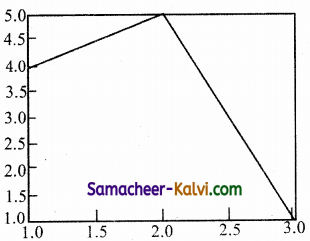(a) import matplotlib.pyplot as pit
plt.plot([l,2,3],[4,5,l])
plt.show( )

(b) import matplotlib.pyplot as pit
plt.plot([l,2],[4,5])
plt.show( )

(c) import matplotlib.pyplot as pit
plt.plot([2,3],[5,l])
plt.show( )

(d) import matplotlib.pyplot as pit
plt.plot([l,3],[4,l])
plt.show( )
(a) import matplotlib.pyplot as pit
plt.plot([l,2,3],[4,5,l])
plt.show( )Question 40.
(a) import matplotlib.pyplot as pit
(b) plt.plot(3, 2)
(c) plt.show( )
Identify the output for the above coding.
(a)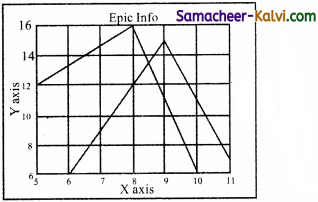(b)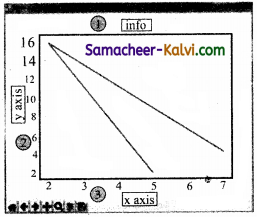(c)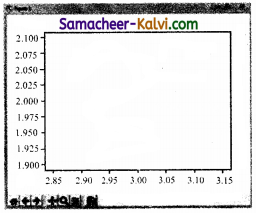(d)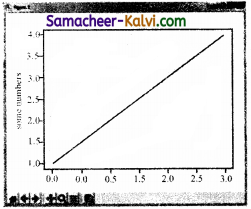(c)Question 41.
Which key is used to run the module?
(a) F6
(b) F4
(c) F3
(d) F5
(d) F5

Question 42.
Identify the right type of chart using the following hints.
Hint 1: This chart is often used to visualize a trend in data over intervals of time.
Hint 2: The line in this type of chart is often drawn chronologically.
(a) Line chart
(b) Bar chart
(c) Pie chart
(d) Scatter plot
(a) Line chartQuestion 43.
Read the statements given below. Identify the right option from the following for pie chart.
Statement A:
To make a pie chart with Matplotlib, we can use the plt.pie( ) function.
Statement B:
The autopct parameter allows us to display the percentage value using
the Python string formatting.
(a) Statement A is correct
(b) Statement B is correct
(c) Both the statements are correct
(d) Both the statements are wrong